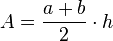# Trapezoid facts for kids

Kids Encyclopedia Facts

A trapezoid (in North America) or trapezium (in Britain and elsewhere) is a quadrilateral, which is defined as a shape with four sides, which has one set of parallel sides. In North America, the term "trapezium" is used for what is elsewhere called an irregular quadrilateral.

## Calculating area

To calculate the area of a trapezoid, you must add the two parallel sides together, divide that by 2, then times that by its height.$A = \frac{a + b}{2} \cdot h$

## Images for kidsTrapezoid Facts for Kids. Kiddle Encyclopedia.